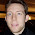## Wednesday, June 04, 2008

### Name of a kind of weight function on a lattice ?

Suppose we have a lattice with elements min, max, and the usual join and meet operations. I define a function w() that maps elements of the lattice to reals, such that
• w(min) = 0
• w(max) = 1
• If x > y, then w(x) > w(y)
• let z = join(x,y). Then w(x) + w(y) >= w(z)
Note that the last condition is slightly weaker than the usual submodularity condition
w(x) + w(y) >= w(z) + w(u) (where u = meet(x,y)).

My question is: is there a well known name for such a weight function ? I've been trying to search various sources, and the closest I came to this was the notion of a 'rank' function for a lattice, which doesn't quite capture this sort-of-metric-property.

1.2.it sounds like a measure function?

3.4.